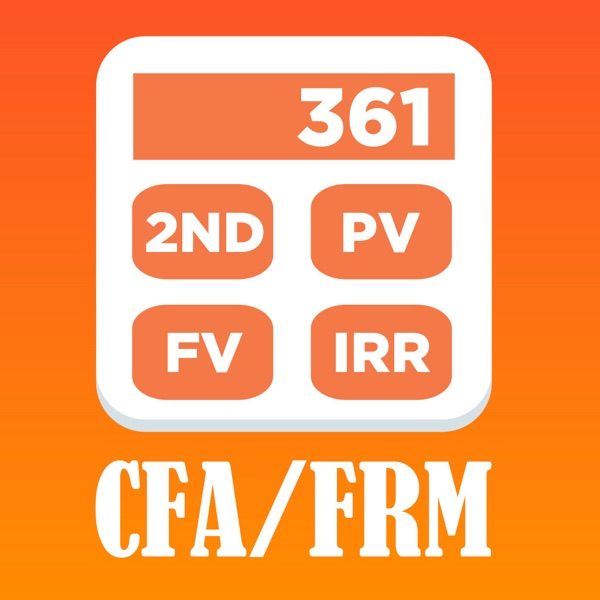BA CFA Calc is a mobile application software based on BA II PLUS calculator. Its use method is consistent with that of physical calculator, which is convenient for CFA/FRM candidates to learn and use.

FULL RANGE OF FINANCE AND SCIENCE FUNCTIONS
• Solves Time-Value-of-Money (TVM) calculations
• Generates amortization schedules
• Performs cash-flow analysis, and computes NPV and IRR
• Calculates depreciation with four different methodologies
• Breakeven, profit and percent difference calculations